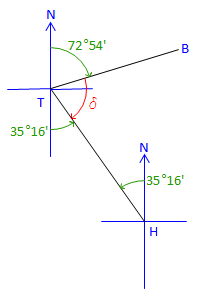## 1. Angles to Directions

Starting with a direction for one line, directions of the other connected lines can be computed from the horizontal angles linking them. The process of addition or subtraction is dependent on the type of horizontal angle (interior, deflection, etc), turn direction (clockwise or counterclockwise), and direction type (bearing or azimuth).

#### a. Examples - Bearing

##### Example 1

The bearing of line GQ is S 42°35' E. The angle right at Q from G to S is 112°40'. What is the bearing of the line QS?

Sketch:Add meridian at Q and label angles:At Q, the bearing to G is N 42°35' W.

Subtracting 42°35' from 112°40' gives the angle, β, from North to the East for line QS.

Bearing QS = N 70°05" E

##### Example 2

The bearing of line LT is N 35°25' W. The angle left at T from L to D is 41°12'. What is the bearing of the line TD?

Sketch:Add meridian at T and label anglesAt T the bearing TL is S 35°25' E.

The bearing angle, σ, is 35°25' + 41°12' = 76°37'

Bearing TD = S 76°37' E.

#### b. Examples - Azimuth

##### Example 1

The azimuth of line WX is 258°13'. At X the deflection angle from W to L is 102°45' L. What is the azimuth of line XL?

Sketch:A deflection angle is measured from the extension of a line. The azimuth of the  extension is the same as that of the line. To compute the next azimuth, the deflection angle is added directly to the previous azimuth.

Because this is a left deflection angle, you would add a negative angle.Azimuth XL = 258°13' + (-102°45') = 155°28'

##### Example 2

The azimuth of line BP is 109°56'. The angle right at P from B to J is 144°06'. What is the azimuth of line PJ?

Sketch:At P, add the meridian and extend the line BPTo get the azimuth of line PB: 109°56' + 180°00'= 289°56'.

Since 144°06' is to the right (+), add it to the azimuth of PB to compute azimuth of PJ: 144°06' + 289°56' = 434°02'Why is the azimuth greater than 360°? Because we've gone past North.

To normalize the azimuth, subtract 360°00': 434°02' - 360°00' = 74°02'

## 2. Directions to Angles

Given directions of two adjacent lines, it is a simple matter to determine the angle between the lines.

#### a. Example - Bearings

The bearing of line HT is N 35°16' W , the bearing of line TB is N 72°54' E. What is the angle right at T from B to H?

Sketch:Label the back-direction at T and angle to be computed, δ.Based on the sketch, the desired angle is what’s left over after both bearing angles are subtracted from 180°00'.

δ = 180°00' - (72°54' + 35°16') = 71°50"

#### b. Example - Azimuths

The azimuth of line MY is 106°12', the azimuth of line YF is 234°06'. What is the angle right at Y from F to M?

Sketch:Label the back-azimuth at Y and angle to be computed, ρ.ρ = 286°12' - 234°06' = 52°06'